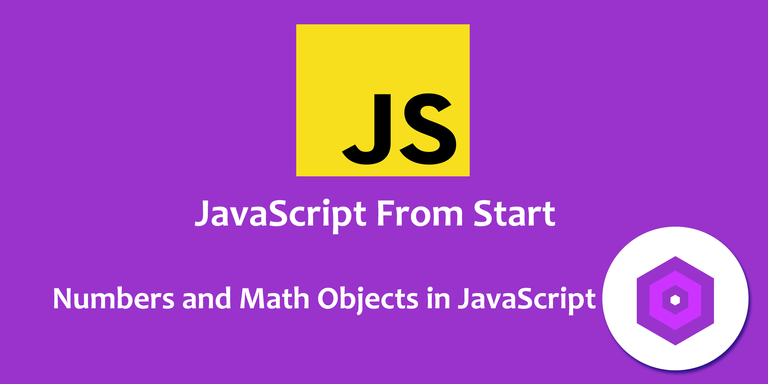# # #JS from Start- Numbers and Math Objects in JavaScript

in GitPlait2 years agoAgain, I got occupied with other things and could not continue the tutorials as planned, but I will ensure I take it to the place I planned.

In the last tutorial, I wrote about Type conversion and Type coercion, and I gave some clear examples and deep explanation about it. Today, we will look at Math object in JavaScript. Unlike, I have been using image to show example, I will write the codes here and you can test them on your editors and check the output as well.

So, let's roll!

``````const fil1 = 80;
const fil2 = 20;

let fil;

Basic math

fil = fil1 + fil2;
fil = fil1 - fil2;
fil = fil1 * fil2;
fil = fil1 / fil2;
fil = fil1 % fil2;

console.log(fil);

``````

Math Objects

``````fil = Math.PI;
``````

Math contains properties and methods. Properties are attributes and method is a function inside an object. PI= 3.141592653589793.

``````fil = Math.E;

This will give us 2.718281828459045
``````

Checking for more helpful methods, we will check round, round up, down and a few.

fil = Math.round(5.8);

.round will round up and down. 5.4 would round down and 5.8 would round up, but you could specify by using. ceil or floor.

Round up

``````fil = Math.ceil(5.4);

This will give 6.

fil = Math.floor(5.8);

This will give 5.

Others are:
fil = Math.sqrt(200);

fil = Math.abs(-4);

Abs is Absolute and the negative number will turn to positive.

fil = Math.pow(10, 2);

100- same as 10*10

fil = Math.min(2,21,89,23,9,-4);

This will output the minimum number which is -4

fil = Math.max(5,99,9,36,2,8);

The Max number is 99
``````

fil = Math.random();

This will give a random numbers and if you want to get a random whole number, you may need to specify.

fil = Math.random() * 30;

29 will be the highest number with decimals. If you want to have 30, you will need to add on 1 and to take way the decimals, you just need to wrap it with Math.floor

fil = Math.floor(Math.random() * 30 + 1);

This is where I will stop today. I hope you get something from it. See you guys on Thursday.

Sort:

Upvoted by GITPLAIT!

We have a curation trial on Hive.vote. you can earn a passive income by delegating to @gitplait
We share 80 % of the curation rewards with the delegators.

To delegate, use the links or adjust 10HIVE, 20HIVE, 50HIVE, 100HIVE, 200HIVE, 500HIVE, 1,000HIVE, 10,000HIVE, 100,000HIVE

Join the Community and chat with us on Discord let’s solve problems & build together.